Next: Tests for the Regression Up: 10.001: Correlation and Regression Previous: Correlation Analysis

# Regression Analysis: Method of Least Squares

Once we have established that a strong correlation exists between x and y, we would like to find suitable coefficients a and b so that we can represent y using a best fit line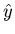= ax + b within the range of the data. The method of least squares is a very common technique used for this purpose. The rationale used here is as follows. For each pair of observations (xi, yi), we define the error ei as

 ei = (axi + b - yi). (2)

Now, we find a and b in such a way that the sum of the squared errors over all the observations is minimized. i.e., the quantity we are interested in minimizing is

 S(a, b) =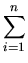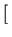axi + b - yi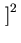. (3)

We know from calculus that to minimize this, we need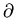S/a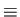0 andS/b0. These conditions yield
 nb +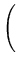xi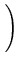a =yixib +xi2a =xiyi. (4)

Eq. 4 gives two linear equations in a and b, which can be solved to get

 a =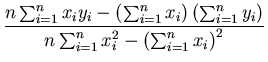, (5)

with b obtained through subsequent substitution of a in either of the two equations given by Eq. 4.

In the case of the data given in Figure 1, the best fit line has a slope of 1.64 and intercept of -0.36. Or in other words,= 1.64x - 0.36. Note that this is only a best fit line which can be used to compute the fuel consumption given the weight within or very close to the range of the measurements. Its predictive power is rather limited. For instance, for x = 0, we get y = - 0.36, which is non-physical. A physical model for the fuel consumption would have predicted 0 consumption of fuel for 0 weight.

How are the slope and the intercept of the best fit line related to the correlation coefficient? To examine this, we rewrite Eq. 5 as

 a ==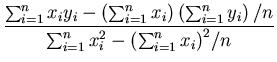=(Verify this step) =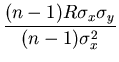(See Eq. 1) = R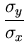. (6)

Similarly, from the first of Eq. 4 and the above result we get

 b =- R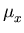, (7)

so that the equation of the best fit line can be represented by=+ (R)(x -). (8)Next: Tests for the Regression Up: 10.001: Correlation and Regression Previous: Correlation Analysis
Michael Zeltkevic
1998-04-15
s for the Regression Up: 10.001: Correlation and Regression Previous: Correlation Analysis
Michael Zeltkevic
1998-04-15